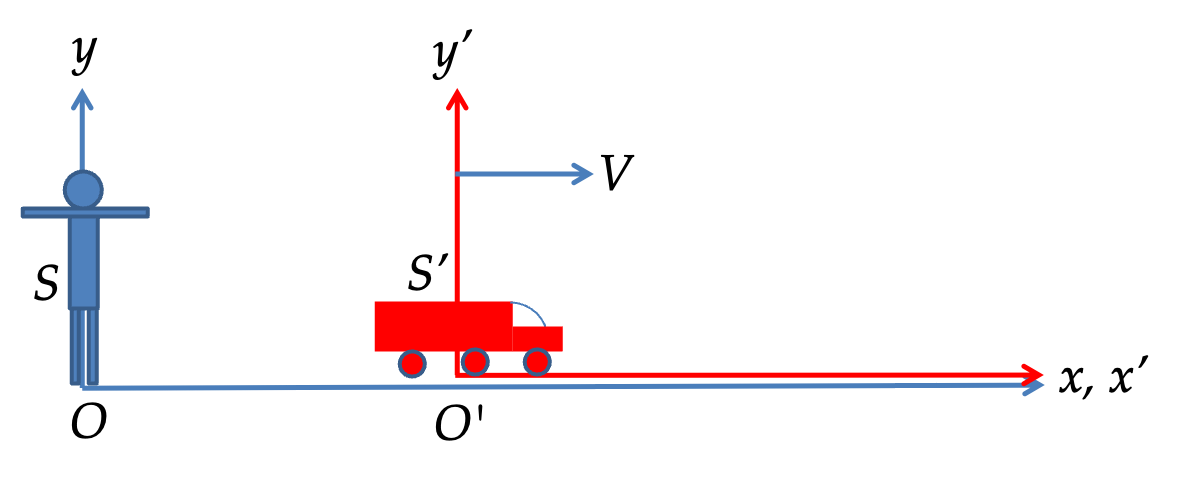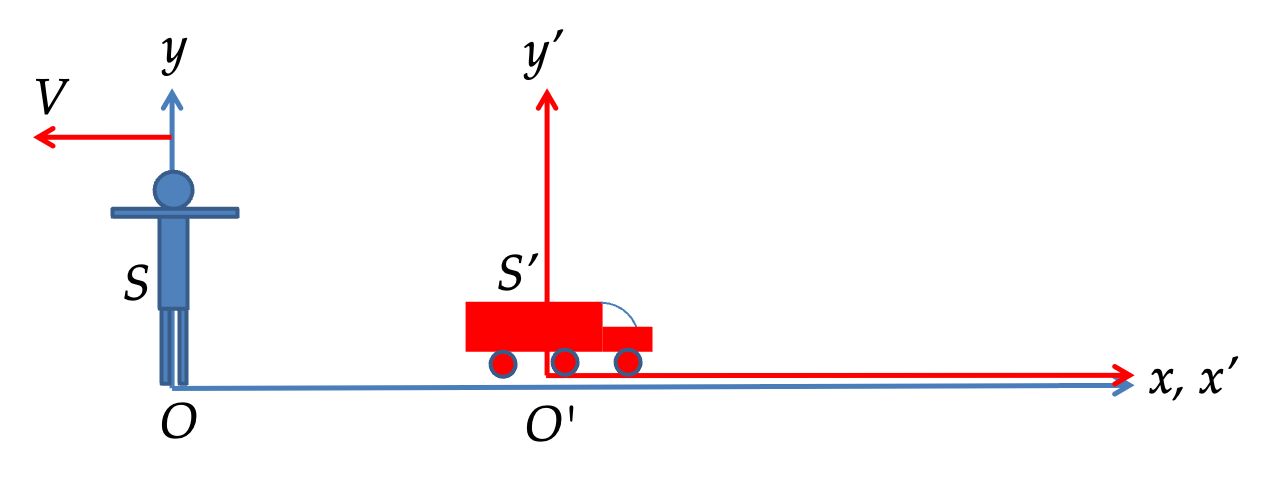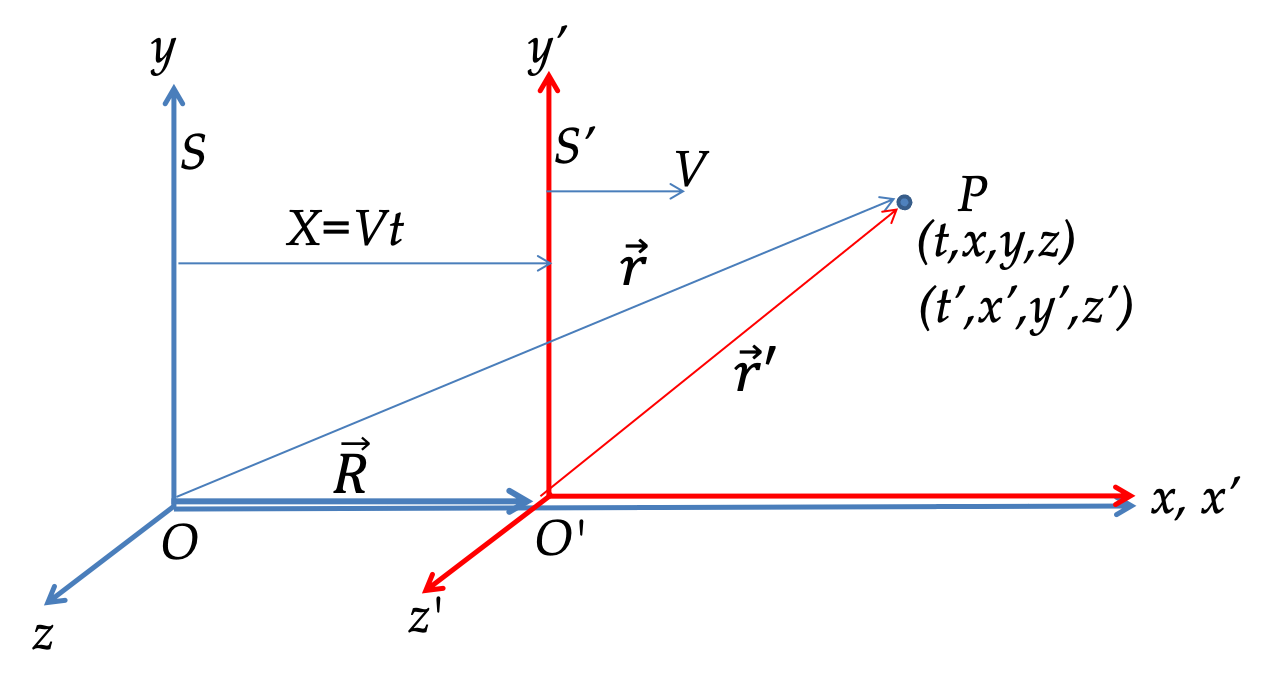## Section52.1Galilean Relativity

The notion of motion is inherently relative. This was perhaps first emphasized by Galileo Galilei. For instance, when you are driving 50 km/h East, the speed of the car with respect to you is actullay just zero since car is not going anywhere with respect to you. By 50 km/h East we mean the car is moving at 50 km/h East with respect to fixed ground.

Not only velocity is relative but so are many other physical quantities such as acceleration, energy, and momentum. For quantities that are relative we would like to know appropriate relation between their values with respect to one reference and corresponding values with respect to another reference frame. Galilean relativity gives us appropriate transformations, given in a section below, when the relative speed of the two reference frames are small compared to speed of light and special relativity applies to speeds upto speed of light.

Any object can be used as a reference with respect to which we can study motion regardless of the state of the motion of the object itself. Even a point in space can be used to define a reference point for this purpose.

We usually attach a set of Cartesian coordinates to the reference to serve as a reference frame. Figure 52.1.1 shows two frames, a frame S with respect to a stationary person and a frame S$^\prime$ which is moving with the truck, which is moving with respect to frame S at speed $V$ as shown.Figure 52.1.1. Two frames S and S$^\prime$ drawn from the perspective of frame S.

It looks weird, but we can redraw Figure 52.1.1 in another way. We can think of truck as stationary. That will make person have velocity $V$ pointed left as shown in Figure 52.1.2. Now, we have frame S$^\prime$ is stationary and frame S moving to the left.Figure 52.1.2. Two frames S and S$^\prime$ drawn from the perspective of frame S$^\prime\text{.}$ This picture is equivalent to Figure 52.1.1.

### Subsection52.1.1Events

In special relativity when you want time in one frame (say, S) from time in another frame (say S$^\prime$), we find that time in S depend on both position and time in S$^\prime\text{.}$ Even though, this is not the case in Galilean relativity, we will carry information of time and position together. This combination, (time, position), is called an event. It refers to some event occuring at the said position at the said time.

### Subsection52.1.2The Galilean Transformations

The Galilean transformations relate the same event in two different frames that are in uniform relative motion with respect to each other. We will look at the position of a particle P from two frame S and S$^\prime$ as shown in Figure 52.1.3, where S$^\prime$ frame is moving towards positive $x$-axis with speed $V$ relative to S.Figure 52.1.3. Coordinates of a particle P with respect to two frames S and S$^\prime\text{.}$

In Galilean relativity, we assume that there is a universal time that can be set to read the same value. That means we will have

\begin{equation} t'=t.\ \ (\textrm{Galilean relativity of time})\label{eq-galilean-relativity-of-time}\tag{52.1.1} \end{equation}

From Figure 52.1.3, it is immediately clear that

\begin{align} \amp x = x' + V t\label{eq-galilean-relativity-of-x}\tag{52.1.2}\\ \amp y = y' \label{eq-galilean-relativity-of-y}\tag{52.1.3}\\ \amp z = z' \label{eq-galilean-relativity-of-z}\tag{52.1.4} \end{align}

These equations are Galilean transformations for the two frames in Figure 52.1.3. By taking time derivatives we can get relations between components of velocity of P in the two frames.

\begin{align} \amp v_x = v'_x + V \label{eq-galilean-relativity-of-vx}\tag{52.1.5}\\ \amp v_y = v'_y \label{eq-galilean-relativity-of-vy}\tag{52.1.6}\\ \amp v_z = v'_z \label{eq-galilean-relativity-of-vz}\tag{52.1.7} \end{align}

Similarly, we get the relation between accceleration of P in the two frames.

\begin{align} \amp a_x = a'_x \label{eq-galilean-relativity-of-ax}\tag{52.1.8}\\ \amp a_y = a'_y \label{eq-galilean-relativity-of-ay}\tag{52.1.9}\\ \amp a_z = a'_z \label{eq-galilean-relativity-of-az}\tag{52.1.10} \end{align}

Since the relative motion between frames is uniform and not accelerating, acceleration will be same in the two frames. Therefore, fixed-mass Newton's law will be same in the two frames.

\begin{equation} \vec F = m \vec a\ \ \longleftrightarrow\ \ \vec F' = m \vec a'.\tag{52.1.11} \end{equation}

This is an illustration of the principle of relativity that state that laws of physics are same for all inertial observers.

We will find below that the Galilean transformations given above depend crucially on the assumption of the existence of an universal absolute time $t\text{.}$ If this assumption is somehow wrong (which it is!!), then all these relations will turn out to be wrong.

A man standing still at a train station watches two boys throwing baseball in a moving train. Suppose the train is moving towards East with a constant speed of $20\text{ m/s}$ and one of the boys throws the ball with a speed of $5\text{ m/s}$ with respect to him towards the other boy who is $5 \text{ m}$ from him towards West. What will be the velocity of the ball as observed by the man on the station.

Hint

Use relative velocity.

$15 \text{ m/s East}\text{.}$

Solution

Let's take positive $x$ axis be pointed towards East. Label quantities with superscript S in the frame of station and with T in the frame of train. From the description of the problem we have

\begin{align*} \amp v_{Tx}^S = + 20\text{ m/s} \ \ \text{(of train in frame of station)}\\ \amp v_{Bx}^T = -5\text{ m/s} \ \ \text{(of ball in frame of train)} \end{align*}

The relation between the two frames gives

\begin{align*} v_{Bx}^S\amp = v_{Tx}^S + v_{Bx}^T \\ \amp = 20 + (-5) = 15\text{ m/s}. \end{align*}

When observed from the Sun at a particular instant Earth and Mars appear to move in the opposite directions with speeds $108,000\text{ km/h}$ and $86,871\text{ km/h}$ respectively. What is the speed of Mars at this instant when observed from Earth?

Hint

Use relative velocity.

$194,871\text{ km/h}\text{.}$

Solution

Let's take positive $x$ axis be pointed in the direction of the motion of Earth at the instant in question. Label quantities with superscript S in the frame of Sun and with E in the frame of Earth. From the description of the problem we have

\begin{align*} \amp v_{Ex}^S = + 108,000\text{ km/h} \ \ \text{(of Earth in frame of Sun)}\\ \amp v_{Mx}^S = -86,871\text{ km/h} \ \ \text{(of Mars in frame of Sun)} \end{align*}

The relation between the two frames gives

\begin{align*} v_{Mx}^E\amp = v_{Mx}^S - v_{Ex}^S \\ \amp = -86,871 - 108,000 = 194,871\text{ km/h}. \end{align*}

A man is running on a straight road perpendicular to a train track and away from the track at a speed of $5\text{ m/s}\text{.}$ The train is moving with a speed of $30\text{ m/s}$ with respect to the track. What is the speed of the man with respect to a passenger sitting at rest in the train?

Hint

Use vector relation.

$30.4\text{ m/s}.$

Solution

Label quantities with superscript G in the frame of ground and with T in the frame of train. Let $x$ axis be pointed in the direction of man's motion and $y$ in the direction of train's motion.

From the description of the problem we have the following components of velocities with respect to the ground.

\begin{align*} \amp v_{mx}^G = 5\text{ m/s} \ \ \text{(of man in frame of Ground)}\\ \amp v_{Ty}^G = 30\text{ m/s} \ \ \text{(of train in frame of Ground)} \end{align*}

Since the velocities in two dimensions, we need to look at vector relation between velocities in different frames.

\begin{equation*} \vec v_{m}^{G} = \vec v_{m}^{T} + \vec v_{T}^{G}. \end{equation*}

Its $x$ and $y$ components will give $x$ and $y$ components of the velocity we desire. Thus,

\begin{align*} \amp v_{mx}^{G} = v_{mx}^{T} + v_{Tx}^{G}, \\ \amp v_{my}^{G} = v_{my}^{T} + v_{Ty}^{G} \end{align*}

give

\begin{align*} \amp v_{mx}^{T} = v_{Tx}^{G} - v_{mx}^{G} = 0 - 5 \\ \amp v_{my}^{T} = v_{Ty}^{G} - v_{my}^{G} = 30 \end{align*}

Therefore, speed of man with respect to train will be

\begin{equation*} v = \sqrt{ (-5)^2 + 30^2 } = 30.4\text{ m/s}. \end{equation*}

A man is running on a straight road that makes $30^\circ$ with the train track. The man is running in the direction on the road that is away from the track at a speed of $5\text{ m/s}\text{.}$ The train is moving with a speed of $30\text{ m/s}$ with respect to the track. What is the speed of the man with respect to a passenger sitting at rest in the train?

Hint

Use vector relation.

$25.8\text{ m/s}\text{.}$

Solution

Label quantities with superscript G in the frame of ground and with T in the frame of train. Let $x$ and $y$ axes be pointed in the direction shown in Figure 52.1.9.

From the description of the problem we have the following components of velocities with respect to the ground.

\begin{align*} \amp v_{mx}^G = 5\cos 60^\circ = 2.5,\ \ v_{my}^G = 5\sin 60^\circ = 4.33 \\ \amp v_{Tx}^G = 0,\ \ v_{Ty}^G = 30 \end{align*}

Since the velocities in two dimensions, we need to look at vector relation between velocities in different frames.

\begin{equation*} \vec v_{m}^{G} = \vec v_{m}^{T} + \vec v_{T}^{G}. \end{equation*}

Its $x$ and $y$ components will give $x$ and $y$ components of the velocity we desire. Thus,

\begin{align*} \amp v_{mx}^{G} = v_{mx}^{T} + v_{Tx}^{G}, \\ \amp v_{my}^{G} = v_{my}^{T} + v_{Ty}^{G} \end{align*}

give

\begin{align*} \amp v_{mx}^{T} = v_{Tx}^{G} - v_{mx}^{G} = 0 - 2.5 \\ \amp v_{my}^{T} = v_{Ty}^{G} - v_{my}^{G} = 30 - 4.33 = 25.67 \end{align*}

Therefore, speed of man with respect to train will be

\begin{equation*} v = \sqrt{ (-2.5)^2 + 25.67^2 } = 25.8\text{ m/s}. \end{equation*}

In a frame at rest with respect to the billiard table, a billiard ball of mass $m$ moving with speed $v$ strikes another billiard ball of mass $m$ at rest. The first ball comes to rest after the collision while the second ball takes off with speed $v$ in the original direction of the motion of the first ball. This shows that momentum is conserved in this frame. (a) Now, describe the same collision from the perspective of a frame that is moving with speed $v$ in the direction of the motion of the first ball. (b) Is the momentum conserved in this frame?

Hint

Drawing figures before and after collision might help.

(a) See below. (b)Yes.

Solution

(a) Let us call the first billiard ball A and the other one B. In the frame that moves with billiard ball A, before the collision this ball will be at rest while ball B will be moving towards ball B with speed $v\text{.}$ After the collision, the frame will continue to move in the same direction as before, but ball A will now move backward with speed $v$ and ball B will be at rest.

(b) Yes, momentum is conserved. Let $+x$ be the direction of motion of A before collision in the ground frame. Then, in the moving frame, we have

\begin{equation*} p_{x}^\text{bef} = p_{Ax}^\text{bef} + p_{Bx}^\text{bef} = 0 +(-mv). \end{equation*}

After the collision

\begin{equation*} p_{x}^\text{aft} = p_{Ax}^\text{aft} + p_{Bx}^\text{aft} = (-mv) + 0. \end{equation*}

In a frame at rest with respect to the billiard table, two billiard balls of same mass $m$ are moving towards each other with the same speed $v\text{.}$ After the collision, the two balls come to rest. (a) Show that momentum is conserved in this frame. (b) Now, describe the same collision from the perspective of a frame that is moving with speed $v$ in the direction of the motion of the first ball. (c) Is the momentum conserved in this frame?

Hint

Drawing figures before and after collision might help.

See solution.

Solution

(a) Let positive $x$ axis point in the first ball. Let's label first ball by A and second ball by B. Looking at the $x$-component of total momentum before and after collision we wee that momentum is conserved, as it should.

\begin{align*} \amp p_{x}^\text{bef} = p_{Ax}^\text{bef} + p_{Bx}^\text{bef} = mv +(-mv) = 0.\\ \amp p_{x}^\text{aft} = p_{Ax}^\text{aft} + p_{Bx}^\text{aft} = 0 + 0 - 0. \end{align*}

(b) In a frame that has same velocity as ball A, ball A will be at rest. Using same direction of positive $x$ axis as in (a) for this frame as well, we find that momenta of the two balls in this frame are

\begin{gather*} p_{Ax}^\text{bef} = 0,\ \ p_{Bx}^\text{bef} = -2mv. \end{gather*}

Hence, total momentum before the collision is

\begin{equation*} p_{x}^\text{bef} = - 2 m v. \end{equation*}

(c) After collision, both balls have $x$-component of velocity equal to $-v$ in this frame. Therefore.

\begin{equation*} p_{x}^\text{aft} = (-mv) + (-mv) = - 2 m v. \end{equation*}

Hence, momentum is conserved in this frame also.